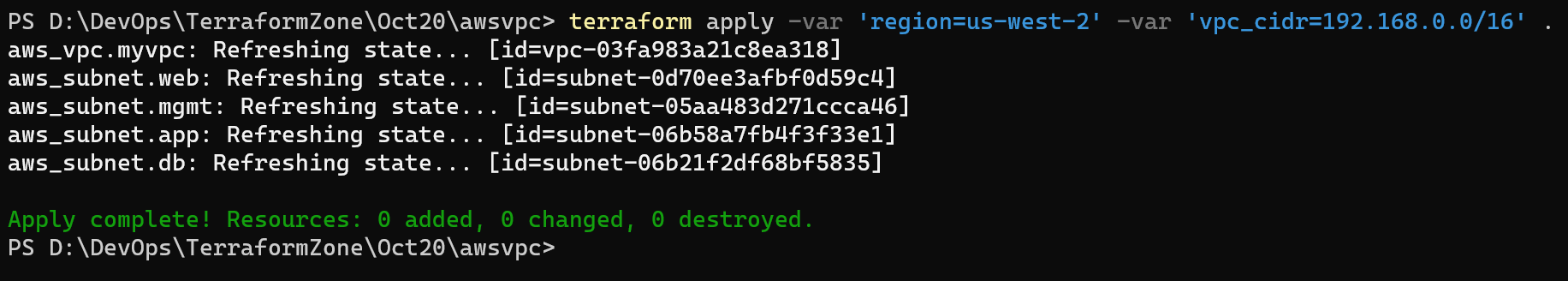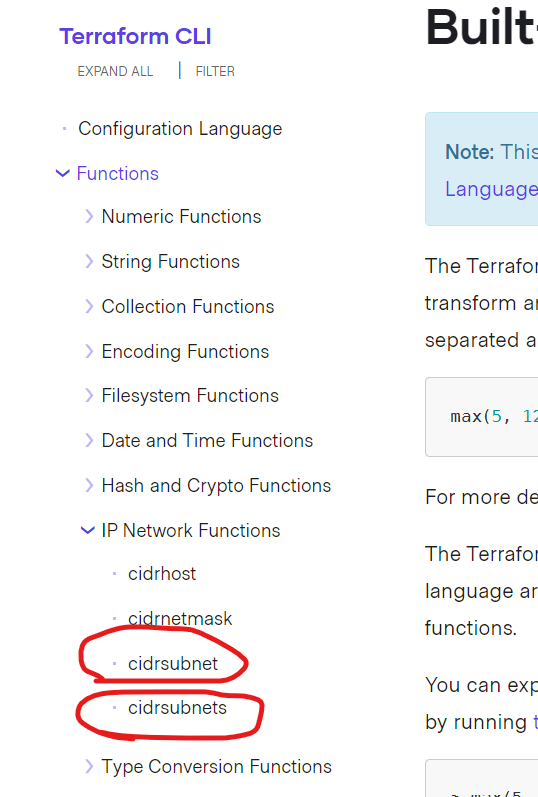### Terraform (contd)

• Third way of Authentication & Authorization in AWS using CLI
• Current state of Terraform Template:
• The template create a vpc with fixed cidr range in a fixed region.
• Lets try to make the template generic
• A variable in a terraform template can accept the input from the user
• for input variables Refer Here
• For the changes done Refer Here• Desigining Varaibles:
• Ensure you have right set of optional variable (Variable with default ) & required variables
• In many cases too many variables is not a good design. If the values can be calculated try calculating them
• To apply above mentioned best practices, lets calculate subnet cidr ranges, for this lets take help from terraform functions Refer Here and expressions Refer Here
• Finding cidrsubnet functions. Refer Here• Refer Here for the changes• For AZs lets use format function of string Refer Here
• Each Region in AWS will have different az’s and lets configure this Refer Here for the changes

This site uses Akismet to reduce spam. Learn how your comment data is processed.